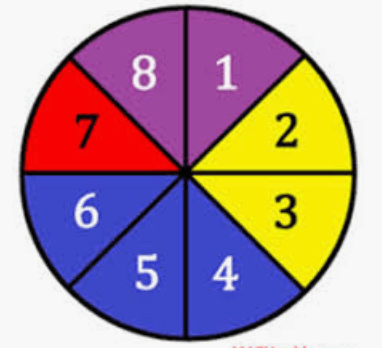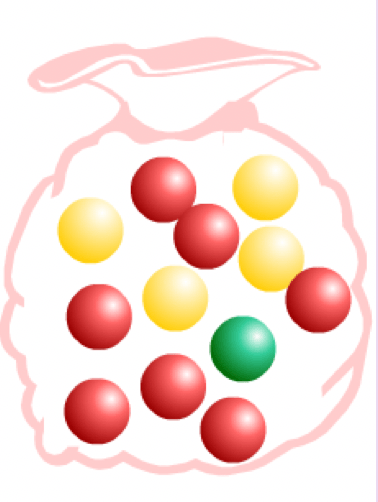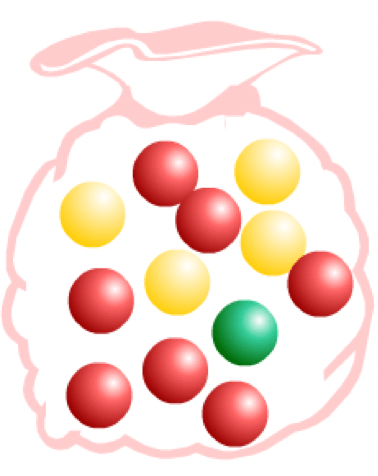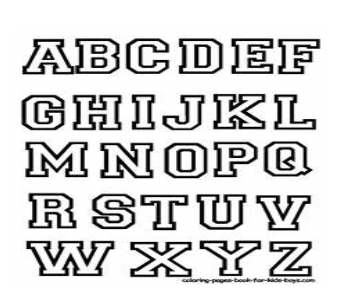Factorials!
Dice
Spinner
Grab Bag
Random Probability
100

5!

What is 120?

100

What is the probability of rolling a four on a number cube? Write as a fraction.

1/6

100

Probability of landing on blue:3/8

100

P(red)=7/12

100

8! ÷ 5!

What is 336?

200

7!

What is 5040?

200

What is the probability of rolling a multiple of 2? Write as a fraction.

3/6

200

Probability of landing on red:1/8

200

P(Green or Yellow) =5/12

200

The probability of you passing this class without turning in any assignments.

What is impossible (0%)?

300

How many ways can 4 students be arranged for a group picture?

What is 4! or 24?

300

What is the probability rolled will be greater than 3?

3/6

300

Probability of not blue:5/8

300

What is the probability of choosing red or green?8/12

300

How many different outcomes are there for rolling two six-sided dice?

What is 36?

400

The sum of 3! and 5!

What is 126?
400

What is the probability as a fraction that the number rolled will be seven?

0/6

400

P(6) =1/8

400

In a bag of marbles, there are 14 swirled, 20 solid colored, and 16 polka dot marbles. What is the probability of grabbing a solid colored marble?

20/50

400

This movie is about children who are selected via lottery to participate in a compulsory televised battle royale to the death.

What is the Hunger Games?

500

In the alphabet what is the probability of selecting a vowel?5/26

Double points

500

What is the probability that the number rolled would be less than or equal to 5?

5/6

500

Probability of multiple of 32/8

500

In a bag of marbles, there are 14 swirled, 20 solid colored, and 16 polka marbles. What is the probability of grabbing a polka marble?

16/50

500

The probability of ending up in a class taught by a male next year (assuming all current teachers return)

What is 50% (1/2, 0.5)

Click to zoom